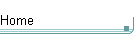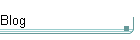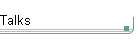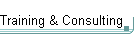GotW #40On theblogNovember 4: Other Concurrency Sessions at PDC November 3 October 26: Hoare on Testing October 23This is the original GotW problem and solution substantially as posted to Usenet. See the book More Exceptional C++ (Addison-Wesley, 2002) for the most current solution to this GotW issue. The solutions in the book have been revised and expanded since their initial appearance in GotW. The book versions also incorporate corrections, new material, and conformance to the final ANSI/ISO C++ standard.

## Controlled PolymorphismDifficulty: 8 / 10

IS-A polymorphism is a very useful tool in OO modeling, but sometimes you may want to restrict which code can use certain classes polymorphically. This issue gives an example, and shows how to get the intended effect.### Problem

1. Consider the following code:

```  class Base {
public:
virtual void VirtFunc();
// ...
};

class Derived : public Base {
public:
void VirtFunc();
// ...
};

void SomeFunc( const Base& );```

There are two other functions. The goal is to allow f1 to use Derived object polymorphically where a Base is expected, yet prevent all other functions (including f2) from doing so.

```  void f1() {
Derived d;
SomeFunc( d ); // works, OK
}

void f2() {
Derived d;
SomeFunc( d ); // we want to prevent this
}```

Demonstrate how to achieve this effect.### Solution

1. Consider the following code:

```  class Base {
public:
virtual void VirtFunc();
// ...
};

class Derived : public Base {
public:
void VirtFunc();
// ...
};

void SomeFunc( const Base& );```

The reason why all code can use Derived objects polymorphically where a Base is expected is because Derived inherits publicly from Base (no surprises here).

If instead Derived inherited privately from Base, then "almost" no code could use Deriveds polymorphically as Bases. The reason for the "almost" is that code with access to the private parts of Derived CAN access the private base classes of Derived and can therefore use Deriveds polymorphically in place of Bases. Normally, only the member functions of Derived have such access. However, we can use "friend" to extend similar access to other outside code.

Putting the pieces together, we get:

There are two other functions. The goal is to allow f1 to use Derived object polymorphically where a Base is expected, yet prevent all other functions (including f2) from doing so.

```  void f1() {
Derived d;
SomeFunc( d ); // works, OK
}

void f2() {
Derived d;
SomeFunc( d ); // we want to prevent this
}```

Demonstrate how to achieve this effect.

```  class Derived : private Base {